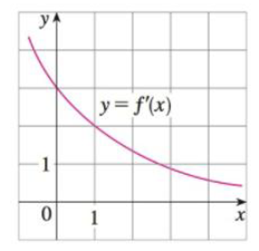Chapter 3.10, Problem 43E

Chapter
Section
Textbook Problem

Suppose that the only information we have about a function f is that f(1) = 5 and the graph of its derivative is as shown.(a) Use a linear approximation to estimate f(0.9) and f(1.1).(b) Are your estimates in part (a) too large or too small? Explain.(a)

To determine

To estimate: The value f(0.9) and  f(0.1).

Explanation

Given:

The value f(1)=5.

Result used:

The linear approximation of the function at x=a is,f(x)f(a)+f(a)(xa).

Calculation:

Obtain the value of f(0.9).

The linearization of the function f(x) at x=0 is computed as follows,

Substitute the value a=1 in f(x)f(a)+f(a)(xa),

f(x)f(1)+f(1)(x1)

Form the given graph, it is observed that f(1)=2

(b)

To determine

To explain: Whether the estimate value in part (a) is too large or too small.

Still sussing out bartleby?

Check out a sample textbook solution.

See a sample solution

The Solution to Your Study Problems

Bartleby provides explanations to thousands of textbook problems written by our experts, many with advanced degrees!

Get Started

Use the guidelines of this section to sketch the curve. y = earctan x

Single Variable Calculus: Early Transcendentals, Volume I

CHECK POINT Let and .Use sets to answer the folowing. 3 .Which pair of is disjoint?

Mathematical Applications for the Management, Life, and Social Sciences

Find the derivative of the function. f(z) = ez/(z1)

Single Variable Calculus: Early Transcendentals

For f(x) = 6x4, f′(x) = _____. 24x 6x3 10x3 24x3

Study Guide for Stewart's Single Variable Calculus: Early Transcendentals, 8th

Find the unit tangent vector for at t = –1.

Study Guide for Stewart's Multivariable Calculus, 8th

What is the primary advantage of self-report measures? What is the primary disadvantage?

Research Methods for the Behavioral Sciences (MindTap Course List)